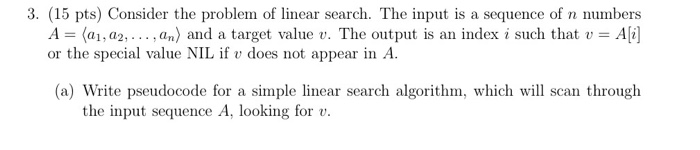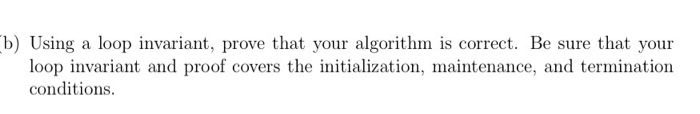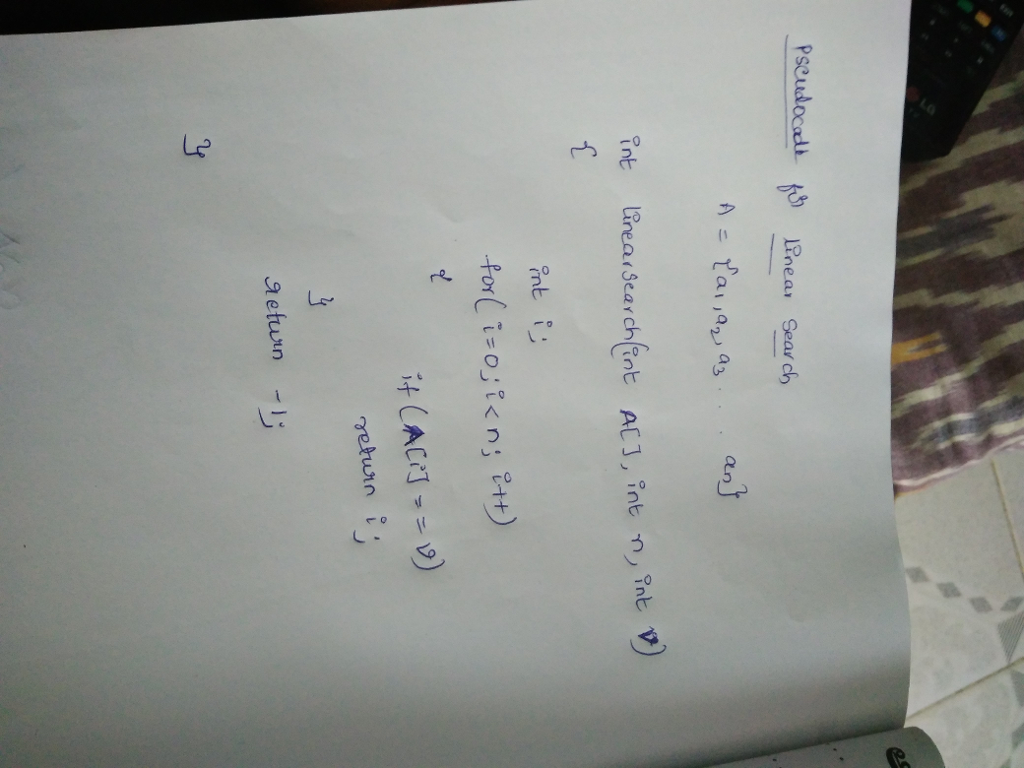# Homework Solution: algorithms…

algorithms3. (15 pts) Consider the problem of linear search. The input is a sequence of n numbers A = 〈a1, a2, . .. , an) and a target value t. The output is an index i such that v = Ali] or the special value NIL if v does not appear in A (a) Write pseudocode for a simple linear search algorithm, which will scan through the input sequence A, looking for v